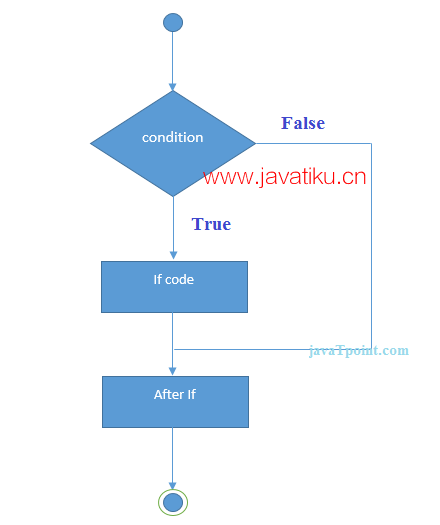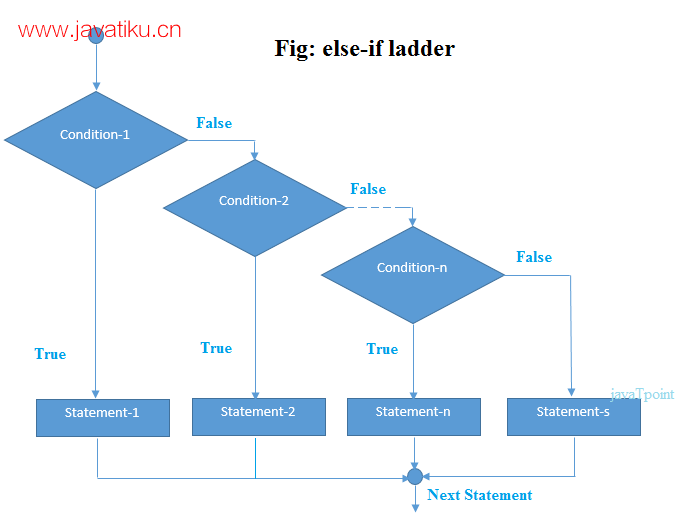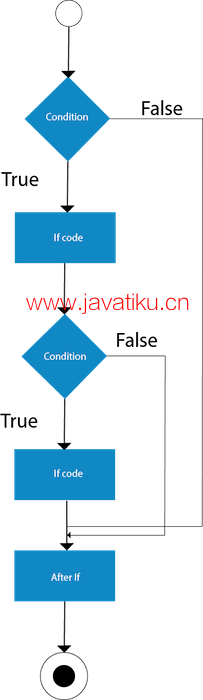# Java教程-详解 Java If-else 语句Java中的if语句用于测试条件，它会检查一个布尔表达式，该表达式的结果可以是true或false。在Java中，有几种类型的if语句可供使用。

1. if语句：if语句是最基本的条件语句，它根据条件的结果来决定执行哪些代码块。
2. if-else语句：if-else语句在if条件为true时执行一个代码块，否则执行另一个代码块。
3. if-else-if阶梯：if-else-if语句允许在多个条件之间进行选择，每个条件都会被逐个检查，直到找到匹配的条件为止。
4. 嵌套if语句：嵌套if语句是if语句的嵌套形式，其中一个if语句包含在另一个if语句内部。这样可以根据多个条件的组合来决定执行哪些代码块。

## Java if 语句

Java if 语句测试条件。如果条件为真，它执行if 块。

``````if(condition){
//要执行的代码
}  ````````````//演示 if 语句使用的 Java 程序
public class IfExample {
public static void main(String[] args) {
//定义一个“年龄”变量
int age=20;
//检查年龄
if(age>18){
System.out.print("年龄大于18");
}
}
}  ``````

``年龄大于18``

## Java if-else 语句

Java if-else 语句也测试条件。如果条件为真，则执行if 块，否则执行else 块

``````if(condition){
//条件为真时的代码
}else{
//条件为假时的代码
}  ````````````//一个Java程序来演示if-else语句的使用。
//这是一个奇数和偶数的程序。
public class IfElseExample {
public static void main(String[] args) {
//定义一个变量
int number=13;
//检查数字是否能被2整除
if(number%2==0){
System.out.println("偶数");
}else{
System.out.println("奇数");
}
}
}  ``````

``奇数``

``````public class LeapYearExample {
public static void main(String[] args) {
int year=2020;
if(((year % 4 ==0) && (year % 100 !=0)) || (year % 400==0)){
System.out.println("闰年");
}
else{
System.out.println("普通年");
}
}
}    ``````

``闰年``

## 使用三元运算符

``````public class IfElseTernaryExample {
public static void main(String[] args) {
int number=13;
//使用三元运算符
String output=(number%2==0)?"even number":"odd number";
System.out.println(output);
}
}  ``````

``odd number``

if-else-if 梯形图语句从多个语句中执行一个条件。

``````if(condition1){
//条件1为真时执行的代码
}else if(condition2){
//条件2为真时执行的代码
}
else if(condition3){
//条件3为真时执行的代码
}
...
else{
//所有条件都为假时执行的代码
}  ````````````//Java程序演示If else-if梯形图的使用。
//它是一个分级系统的程序，不及格，D级，C级，B级，A级和A+。
public class IfElseIfExample {
public static void main(String[] args) {
int marks=65;

if(marks<50){
System.out.println("fail");
}
else if(marks>=50 && marks<60){
}
else if(marks>=60 && marks<70){
}
else if(marks>=70 && marks<80){
}
else if(marks>=80 && marks<90){
}else if(marks>=90 && marks<100){
}else{
System.out.println("Invalid!");
}
}
}  ``````

``C grade``

``````public class PositiveNegativeExample {
public static void main(String[] args) {
int number=-13;
if(number>0){
System.out.println("POSITIVE");
}else if(number<0){
System.out.println("NEGATIVE");
}else{
System.out.println("ZERO");
}
}
}    ``````

``NEGATIVE``

## Java嵌套if语句

``````if(condition){
//要执行的代码
if(condition){
//要执行的代码
}
}  ````````````//Java程序演示嵌套If语句的使用。
public class JavaNestedIfExample {
public static void main(String[] args) {
//为年龄和体重创建两个变量
int age=20;
int weight=80;
//applying condition on age and weight
if(age>=18){
if(weight>50){
System.out.println("您符合献血条件");
}
}
}}  ``````

``您符合献血条件``

``````//Java程序演示嵌套If语句的使用。
public class JavaNestedIfExample2 {
public static void main(String[] args) {
//为年龄和体重创建两个变量
int age=25;
int weight=48;
//在年龄和体重上应用条件
if(age>=18){
if(weight>50){
System.out.println("您符合献血条件");
} else{
System.out.println("您不符合献血条件");
}
} else{
System.out.println("年龄必须大于18岁");
}
}  }  ``````

``您不符合献血条件``